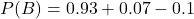There are two known issues with a certain model of new car. The first issue, A, occurs with a probability of P(A)=0.1. B is another known is

Question

There are two known issues with a certain model of new car. The first issue, A, occurs with a probability of P(A)=0.1. B is another known issue with the car. If it is known that either event occurs with a probability of P(A OR B)=0.93, and that both events occur with a probability of P(A AND B)=0.07, calculate P(B).

in progress 0
5 months 2021-08-10T02:09:23+00:00 1 Answers 40 views 0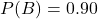Step-by-step explanation:

Given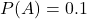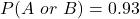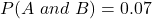Required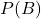To do this, we make use of: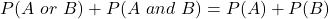Make P(B) the subject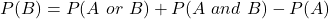So, we have: